# Oberseminar Analysis Winter 2016/17

## Organizers: C. Seis, S. Conti, H. Koch, S. Müller, B. Niethammer, M. Rumpf, C. Thiele, J.J.L. Velázquez

• Thursday, October 27, 2:15 p.m., Lipschitz-Saal
Sebastian Throm (U Bonn)
Uniqueness of self-similar profiles for Smoluchowski's equation
Smoluchowski's coagulation equation is a kinetic model which is used to describe aggregation processes arising in a large number of different applications from physics, chemistry and biology. Experimental and numerical considerations suggest that for large times, the solutions to this model have a self-similar form. This is known as the 'scaling hypothesis'.
In this talk we address the question of uniqueness of such self-similar profiles and we present a first rigorous result for perturbations of an explicitly solvable model.

• Thursday, November 3, 2:15 p.m., Lipschitz-Saal
Mircea Petrache (MPI Bonn)
Three types of vortices and the associated notions of optimal transport
Vortices and topological singularities appear naturally in variational problems from physics and geometry. In particular this is the case when the function spaces which are natural for a given theory or model, contain objects (which could be vector fields, or maps, or connections on bundles) of regularity low enough that Sobolev embeddings into spaces of continuous objects do not hold.
I will briefly present results concerning the classical study of Sobolev maps $W^{1,2}(\mathbb R^3, \mathbb S^2)$, for which the model-singularity is the unit vector field $V(x)=x/|x|$ with a singularity "of degree one" at the origin, for which the lack of approximability by smooth maps is encoded in a "minimal connection", enterpretable as a discrete optimal transport plan, between singularities.
I then consider three other types of singularities which appear in gauge theory and for different spaces of Sobolev maps, where the replacement of the above classical discrete optimal transport is either
a) a functional on ensembles of paths having a constraint on the distribution of endpoints (partly done in projects in collaboration with L. Brasco and T. Riviere)
b) a version of discrete optimal transport "modulo 2" (studied in collaboration with R. Zuest)
c) branched transport (appearing in a recent result of F. Bethuel).

• Thursday, November 10, 2:15 p.m., Lipschitz-Saal
David Fajman (U Wien)
Decay Estimates for Geometric Transport Equations
Transport equations appear in numerous models in mathematical physics. In General Relativity, they are relevant in the context of the Einstein-Vlasov system (EVS) modeling spacetimes with ensembles of self-gravitating, collisionless particles. Understanding the long-time behavior of solutions to the EVS requires analytic methods to control the energy-density of Vlasov matter in spacetimes of non-trivial geometry both in the asymptotically flat case as well as on spacetimes which are spatially compact. We present two methods to control Sobolev-type energies of the Vlasov distribution function in these respective geometric scenarios. The first approach is a generalized vector field method for transport equations that has been developed in collaboration with J. Smulevici (Paris) and J. Joudioux (Vienna). The second approach concerns L^2-Sobolev-type estimates for distribution functions on closed manifolds of time-dependent geometry. We finally comment on full non-linear stability problems that have been resolved by these methods.

• Thursday, November 17, 2:15 p.m., Lipschitz-Saal
Emanuel Indrei (Purdue U)
The Tangential Touch Problem for Fully Nonlinear Elliptic Operators
The tangential touch problem in elliptic theory consists of exposing the dynamics of the free boundary near the fixed boundary in obstacle problems. The solution of this problem is discussed for fully nonlinear elliptic operators in two dimensions. Based on joint work with Andreas Minne.

• Thursday, November 24, 2:15 p.m., room 0.008
Elmar Schrohe (U Hannover)
The porous medium equation on manifolds with conical singularities
The porous medium equation is the quasilinear diffusion equation $$u'(t)-\Delta(u^{m}(t))=f(u,t),\ t\in(0,T_0],$$ with initial condition $u(0)=u_{0}$. It describes the flow of a gas in a porous medium; here $u$ is the density of the gas, $m>0$ and $f$ is a forcing term which we assume for simplicity holomorphic in $u$ and Lipschitz in $t$.
We study this equation on a manifold with conical singularities which we model by an $n$-dimensional compact manifold with boundary, $n>1$, endowed with a degenerate Riemannian metric $g$, which, in a collar neighborhood $[0,1)\times\partial{{\mathbb B}}$ of the boundary $\partial{{\mathbb B}}$, is of the form $g(x,y)=dx^{2}+x^{2}h(y)$ for a (non-degenerate) Riemannian metric $h$ on $\partial{{\mathbb B}}$. By $\Delta$ we denote the Laplacian associated with this metric; it naturally acts on scales of weighted Mellin-Sobolev spaces $\mathcal{H}^{s,\gamma}_p({{\mathbb B}})$.
Whenever the initial value $u_0$ is strictly positive, we can show existence, uniqueness and maximal $L^{p}$-regularity of a short time solution. In particular, we obtain information on the short time asymptotics of the solution near the tip of the cone.
Actually, these solutions turn out to be instantaneously smooth for positive time outside the conic singularity. I also report on progress towards establishing the long time existence of maximal regularity solutions when the initial data are positive and the forcing term $f$ is zero and the existence of weak solutions for the case of non-negative initial data and zero forcing term.

• Thursday, December 1, 2:15 p.m., Lipschitz-Saal
Franz Gmeineder (U Oxford)
Regularity Theory for Convex Functionals of Linear Growth
In this talk we present recent results on the regularity theory for convex functionals of linear growth. Depending on whether the full or merely the symmetric gradients appear in such functionals, we give conditions on the variational integrals to produce minimisers of higher Sobolev- or Hölder regularity within the framework of Dirichlet and Neumann problems, respectively. This talk comprises joint work with L. Beck (Augsburg), M. Bulicek (Prague) and J. Kristensen (Oxford).

• Thursday, December 8, 2:15 p.m., Lipschitz-Saal
Sebastian Schwarzacher (Charles U)
Very weak solutions to non-Newtonian fluids
I will present some recent works concerning the existence, uniqueness and optimal regularity for a class of very weak solutions to certain non-linear partial differential equations. The theory covers some models of non-Newtonian fluids including liquids or gases which change their viscose behavior with respect to their shear speeds. We will introduce some structural constraints on the viscosity which are sufficient and to some extend necessary to built a unified existence and uniqueness theory: Our approach allows to prove qualitatively the same so called L^p-theory for our models as is known to be satisfied by the Poisson equation.

• Thursday, December 15, 2:15 p.m., Lipschitz-Saal
Eris Runa (MPI Leipzig)
On the optimality of periodic stripes in a continuous variational model with non-local interaction.
In this talk, we will discuss the continuous version of a variational problem proposed by Giuliani, Lebowitz and Lieb. The model has a perimeter term and a non-local term, which are in competition. We compute the $\Gamma$-limit as the parameter $J$ converges to its critical value $J_c$. The $\Gamma$-limit will turn out to be finite only on stripes. By using the convergence of the minimizers, we show that minimizers converges to periodic stripes. This work is in collaboration with Michael Goldman.

• Thursday, January 5, 2:15 p.m., Lipschitz-Saal
Duy Phan-Duc (Radon Institute Linz)
Stabilization to trajectories for parabolic equations
The exponential stabilization to trajectories problem is addressed for a general class of semilinear parabolic systems. The controls take values in a finite-dimensional space. Both internal and boundary actuators are supported in small region. We prove that under suitable conditions on the family of actuators, they allow us to stabilize the system. Moreover, some estimates on the number of actuators that we need to stabilize the system are established. Using the Dynamical Programming Principle, the stabilizing controls can be taken in feedback form and be computed by solving a suitable differential Riccati equation. Some simulations are presented by using Finite Element Method (FEM) and performed with MATLAB.

• Thursday, January 12, 2:15 p.m., room 0.008
Illya M. Karabash (U Bonn and IAMM of NAS of Ukraine)
Pareto optimization of resonances in layered media and Krein strings.
Resonators with small decay rate are required in Optical Engineering because they enhance intrinsically small light-matter interactions. Since light is difficult to localize, it is hard to realize small-sized cavities with strong light confinement (high-Q resonators). Mathematically, the problem is to design, under certain constraints, a structure of the medium in such a way that it generates a resonance as close as possible to the real line. While engineering and computational aspects of fabrication of high-Q resonators have drawn great attention during the last decade, the analytic background of spectral optimization for non-Hermitian eigenproblems is still in the stage of development. The goal of the talk is to present a rigorous approach to resonance optimization. The fact that resonances move in the complex plane brings to the problem the features of Pareto optimization and makes it necessary to develop a multi-parameter approach to the perturbation theory.

• Thursday, January 19, 2:15 p.m., Lipschitz-Saal
Ben Wallace (UBC Vancouver)
Finite-order correlation length of the $|\varphi|^4$ spin model in four dimensions
The correlation length of order p for the $|\varphi|^4$ spin model (a continuous-spin version of the O(n) model) is a normalization of the p-th moment of its two-point function. We will outline the proof (based on a renormalisation group method of Bauerschmidt, Brydges, and Slade) that, in the upper-critical dimension 4, the correlation length of order p undergoes mean-field scaling with a logarithmic correction as the critical point for this model is approached from above (for sufficiently weak coupling). Via a supersymmetric integral representation, this result also extends to the weakly self-avoiding walk with a contact attraction, for which the correlation length of order p is closely related to the mean p-th displacement of the walk. This is joint work with Roland Bauerschmidt, Gordon Slade, and Alexandre Tomberg.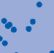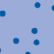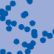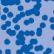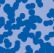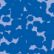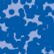## News

Den Hausdorff Memorial Prize für die beste PhD-Arbeit 2018 der Fachgruppe Mathematik erhielt Frau Eva Kopfer aus der Stochastischen Analysis (Prof. Dr. T. Sturm). (26.01.2019)

Einen der BMG-Bachelor-Preise 2018 für die beste Bachelor-Arbeit hat Herr Adrian Riekert für seine Arbeit "Statistical Mechanics of a Two-Dimensional Inviscid Fluid" bei Prof. Dr. M. Gubinelli erhalten. (23.01.2019)

Frau Dr. Antje Kiesel hat mit zwei weiteren Partnern einen Lehr-Sonderpreis der Fakultät 2018 für das e-learning Projekt "Fit4Math" erhalten.

The Department of Mathematics and the Hausdorff Center for Mathematics of the University of Bonn mourn over Prof. Dr. Kazumasa Kuwada. (more)

The Universidad Politécnica de Madrid (UPM) has awarded Professor Michael Ortiz a Doctorate Honoris Causa (honorary doctorate). (10.12.2018)

Prof. Stefan Müller has been elected as Fellow of the European Academy of Sciences (15.10.2018).

Prof. Sergio Albeverio was awarded on 28/09/2018 the title Doctor Honoris Causa
at the Dept. of Mathematics, Stockholm University. (08.10.2018)

Contact

Managing Director: Prof. Dr. Massimiliano Gubinelli
Chief Administrator: Dr. B. Doerffel
geschaeftsfuehrung@iam.uni-bonn.de
Imprint | Datenschutzerklärung